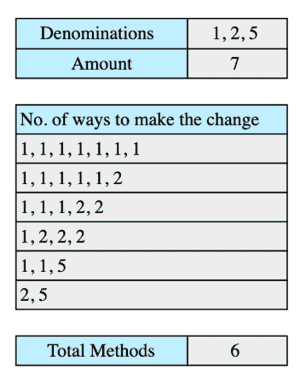# Coin Changing Problem

## Problem Statement

Suppose we have coin denominations of [1, 2, 5] and the total amount is 7. We can make changes in the following 6 ways:## Hint

• Use principles of dynamic programming

## Try it yourself

int solve_coin_change(vector<int>& denominations, int amount) {
// TODO: Write - Your - Code
return -1;
}

## Solution

int solve_coin_change(vector<int>& denominations, int amount) {

vector<int> solution(amount + 1);
solution = 1;

for (int den: denominations) {
for (int i = den; i < (amount + 1); ++i) {
solution[i] += solution[i - den];
}
}

return solution.back();
}

int main() {
vector<int> denominations = {1, 2, 5};
int amount = 7;
int result = solve_coin_change(denominations, amount);
// printing result
cout<< "solve_coin_change_dp([";
for (int den: denominations){
cout << den << " ";
}
cout << "], " << amount << ") = " << result;
}

## Solution Explanation

### Runtime complexity

The runtime complexity of this solution is quadratic, O(m×n), where m is the number of denominations and n is the total amount.

### Memory complexity

The memory complexity of this solution is linear, O(n), where n is the total amount.

### Solution Breakdown

To apply a dynamic programming approach, the problem to be solved must have optimal substructure. Optimal structure means that the optimal solution of the problem at hand could be achieved by leveraging the optimal solutions of its subproblems and overlapping sub-problems. One way to achieve this is by using pre-computed values for solved sub-problems so that we don’t have to solve them again.

The coin changing problem has both optimal substructure, meaning that it can be easily divided to simpler problems and they can be solved to find the final solution. It also satisfies the property of overlapping sub problems, meaning previously solved sub problem results can be used multiple times.

To solve this problem, we’ll keep an array of size amount + 1. One additional space is reserved because we also want to store the solution for the 0 amount.

There is only one way you can make a change of 0, i.e., select no coin so we’ll initialize solution = 1. We’ll solve the problem for each amount, denomination to amount, using coins up to a denomination, den.

The results of different denominations should be stored in the array solution.

The solution for amount x using a denomination den will then be:

solution[x] = solution[x] + solution[x - den]


We’ll repeat this process for all the denominations, and at the last element of the solution array, we will have the solution.

Considering the example mentioned above, the code works in the following way:

• Initially solution = 1 and solution[x] = 0 for all x > 0 and x <= amount.

• We’ll start with first denomination which is 1 in our example, and will update solution[x] = solution[x] + solution[x - 1] for all 1 <= x <= amount.

• Now, we’ll use second denomination, i.e. 2, in our example. We’ll compute solution[x] = solution[x] + solution[x - 2] for all 2 <= x <= amount.

• At the end, we’ll use third denomination, i.e. 5, in our example. We’ll compute solution[x] = solution[x] + solution[x - 5] for all 5 <= x <= amount.

Learn in-demand tech skills in half the time